• 自己写的傅里叶变换函数，带绘图，时域、频域对比。 输入参数data，时域信号。 Fs采样频率需根据实际修改。
• 中国科技论文在线 基于 MATLAB GUI 的傅里叶变换分析的仿 真设计 高恒伟宋余君何辉* 中国矿业大学信电学院江苏徐州 221008 摘要本文简要阐述了傅里叶变换的基本原理傅里叶变换的性质傅里叶变换的尺度变换 性频移特性...
• 不过快速傅里叶变换和数论变换不是一两句话可以口胡明白的，也不是本文想讲的知识，姑且略去…… 最后，如果自己手写的FFT而没用complex库里的复数运算的话，这题卡精度，要用long double。 诸君来看代码吧： #...


I have a set of super poker cards, consisting of an inﬁnite number of cards. For each positive composite integer p, there are exactly four cards whose value is p: Spade(S), Heart(H), Club(C) and Diamond(D).There are no cards of other values.我有一副超级扑克包含无数张牌，如果数字是正合数的话，那就有四种牌：黑桃(S)、红桃(H)、梅花(C)、方片(D)。By “composite integer”, we mean integers that have more than 2 divisors. For example, 6 is a composite integer, since it has 4 divisors: 1, 2, 3, 6; 7 is not a composite number, since 7 only has 2 divisors: 1 and 7. Note that 1 is not composite (it has only 1 divisor). 比如6有4个因子(1，2，3，6)所以它是合数；7只有两个(1，7)那就不是合数。Given a positive integer n, how many ways can you pick up exactly one card from each suit (i.e. exactly one spade card, one heart card, one club card and one diamond card), so that the card values sum to n? For example, if n = 24, one way is 4S + 6H + 4C + 10D, shown below:给定一个整数n，从四种花色各选一张牌，使得四张牌点数和为n，求解有多少种选法组合。比如n=24时，其中的一种选法可以为24=4+6+4+10，如下图所示：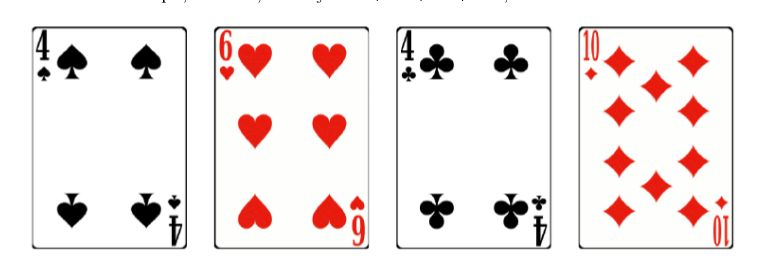Unfortunately, some of the cards are lost, but this makes the problem more interesting. To further make the problem even more interesting (and challenging!), I’ll give you two other positive integers a and b, and you need to ﬁnd out all the answers for n = a, n = a + 1, ..., n = b.不幸的是有的牌丢失了，样例会给出哪些丢了。并且为了题目更加因吹斯听，实际上不是给你n，而是给两个数a、b，输出所有n=a、n=a+1、n=a+2、……、n=b时的答案。InputThe input contains at most 25 test cases. Each test case begins with 3 integers a, b and c, where c is the number of lost cards. The next line contains c strings, representing the lost cards. Each card is formatted as valueS, valueH, valueC or valueD, where value is a composite integer. No two lost cards are the same. The input is terminated by a = b = c = 0. There will be at most one test case where a = 1, b = 50,000 and c ≤ 10,000. For other test cases, 1 ≤ a ≤ b ≤ 100, 0 ≤ c ≤ 10.输入有多组数据，每组数据第一行三个整数a、b、c，a、b如上，c为丢失卡牌数；第二行给出c个具体的卡牌为丢失的。abc均为0时输入结束。OutputFor each test case, print p integers, one in each line. Print a blank line after each test case.输出如题~记得空行Sample Input12 20 24S 6H0 0 0Sample Output000000103拜早年啦！年夜是刷题，还是边看番边刷题，还是带亲戚家小孩刷题啊？口胡思路：先以只有两色四张牌的简单题为例。假如第一个花色S有4和6两个点数，则可以用一个多项式表示所有可选情况：x^4 + x^6；第二个花色H有8和10两个点数，可用：x^8 + x^10表示。我们将这两式相乘得(x^4 + x^6)(x^8 + x^10) = x^12 + 2 * x^14 + x^16，这个结果代表的意义就是：和为12的组合有1种，和为14的组合有2种(4S+10H或6S+8H)，和为16的有1种。好，那么对应到最开始的这道题目上来，就是稍微复杂了一点。对于每个花色，x的次幂为质数时系数为0，即多项式中只存在合数项。然后合数中也会有丢失的，其系数也置零。接下来四个多项式相乘即可，最终的多项式中x的次幂为n的项的系数，就是和为n的组合数。事实上，这是离散数学中的一个小知识点，如题，是生成函数的一个简单应用，在此处帮大家复习一下。另外，两多项式相乘的朴素做法显然是O(n^2)的，用FFT或者NTT加速成nlogn喽。不过快速傅里叶变换和数论变换不是一两句话可以口胡明白的，也不是本文想讲的知识，姑且略去……最后，如果自己手写的FFT而没用complex库里的复数运算的话，这题卡精度，要用long double。诸君来看代码吧：#include #include #include #include using namespace std;const int maxn = 50010;const long double PI = acos(-1.0);struct Complex {    long double x, y;    Complex(long double a = 0, long double b = 0):x(a), y(b){}};//手写复数运算Complex operator + (Complex A, Complex B) { return Complex(A.x + B.x, A.y + B.y); }Complex operator - (Complex A, Complex B) { return Complex(A.x - B.x, A.y - B.y); }Complex operator * (Complex A, Complex B) { return Complex(A.x * B.x - A.y * B.y, A.x * B.y + A.y * B.x); }Complex n[maxn * 4], m[maxn * 4];//像线段树一样要开4倍int r[maxn * 4], l, limit, nl;/*---以上为FFT所需部分---*/int a, b, c;//abc含义见题面bool composite[maxn];//合数记录bool lost[maxn];//卡牌丢失记录void init(int n) {//求50000以内的合数    int m = int(sqrt(n) + 0.5);    for (int i = 2; i <= m; i++)        if (!composite[i])            for (int j = i * i; j <= n; j += i)                composite[j] = 1;}const char *ss = "SHCD";int idx(char c) {//巧得丢失字符位置    return strchr(ss, c) - ss;}/*--以下为一个普通的FFT板子--*/void fft_init() {    limit = 1, l = 0;    while (limit 2*(b + 1)) {        limit <<= 1;        l++;    }    for (int i = 0; i         r[i] = (r[i>>1] >> 1) | ((i&1) <-1));}void fft(Complex *A, int type) {    for (int i = 0; i         if (i             swap(A[i], A[r[i]]);    for (int mid = 1; mid 1) {        Complex Wn(cos(PI / mid), type * sin(PI / mid));        for (int R = mid <1, j = 0; j             Complex w(1, 0);            for (int k = 0; k                 Complex x = A[j+k], y = w * A[j+k+mid];                A[j+k] = x + y;                A[j+k+mid] = x - y;            }        }    }}void Fourier(Complex *A, Complex *B) {    fft(A, 1);    fft(B, 1);    for (int i = 0; i         A[i] = A[i] * B[i];    fft(A, -1);    for (int i = 0; i 1; i++)        A[i].x /= limit, A[i].y = 0.0;}/*---FFT结束---*/int main() {    init(50000);//求5万以内合数    while (~scanf("%d%d%d", &a, &b, &c) && a) {        memset(lost, false, sizeof(lost));//丢牌数组        for (int i = 0; i             int temp; char ch;            scanf("%d%c", &temp, &ch);            lost[idx(ch)][temp] = 1;//这张牌丢了        }        n.x = 1.0, n.y = 0.0;//同下方m数组注释        nl = 1;//常数项        fft_init();//这个东西反复调用没意义就先拿出来了        for (int i = 0; i 4; i++) {            //n是上一轮结束时的多项式结果，nl是多项式n的长度            //答案只要求a到b的多项式系数，所以次幂为b以上可以置零            for (int j = nl; j <= limit; j++)                n[j].x = n[j].y = 0.0;            //m数组用来表示当前花色的多项式，所以先全置零            for (int j = 0; j <= limit; j++)                m[j].x = m[j].y = 0.0;            for (int j = 4; j <= b; j++) {                if (composite[j] && !lost[i][j])                    m[j].x = 1.0, m[j].y = 0.0;                    //将这个合数的多项式系数设为复数(1 + 0*i)，即实数的1            }            Fourier(n, m);//n和m这两个多项式相乘            nl = b + 1;//b+1的原因是常数项也是一项        }        for (int i = a; i <= b; i++)//x为实数项，即系数            printf("%lld\n", (long long)(n[i].x + 0.5));        puts("");    }    return 0;}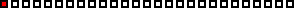温馨提示如果你喜欢本文，请分享到朋友圈，想要获得更多信息，请关注ACM算法日常。点赞的时候，请宠溺一点

展开全文常用函数的傅里叶变换
• WORD格式 专业资料整理 MATLAB作业 方法一 N=200;dt=0.001;n=1:200; x=3*sin(2*pi*10*n*dt)+3*sin(2*pi*30*n*dt)+sin(2*pi*40*n*dt)+sin(2 *pi*50*n*dt)+sin(2*pi*60*n*dt)+6*sin(2*pi*80*n*dt; %建立时间序列 X=...
• 今天，二狗给大家讲一讲Matlab实现傅里叶变换。大家都知道，信号分为两种，确定信号和不确定信号。在确定信号中，有两个非常重要的类别，时域分析和频域分析。而将两者充分结合的，就是我们今天要讲的傅里叶变换。绝...


嗨，大家好。二狗又回来了。今天，二狗给大家讲一讲Matlab实现傅里叶变换。大家都知道，信号分为两种，确定信号和不确定信号。在确定信号中，有两个非常重要的类别，时域分析和频域分析。而将两者充分结合的，就是我们今天要讲的傅里叶变换。绝大多数工科狗在大一或者大二的时候，都或多或少接触过傅里叶变换。二狗也不例外。当初二狗学《复变函数与积分变换》时，差点被搞成死狗，就是因为傅里叶变换。下面这个三维图就是频域和时域上的周期函数，非常形象的显示了这个道理。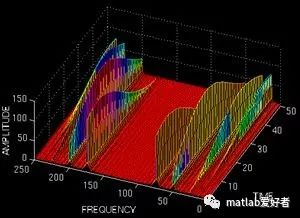由上可知，在空间中。从频率或者时域的角度都可以描述这个周期函数。下面，我们先帮助大家简单回忆一下傅里叶变换的基本原理。周期函数都是时域表达式，既然有周期，当然也就有频率。有些时候，我们需要用到函数的频域表达式。这就需要在频域和时域之间转化。最简单的就是利用周期函数的三角函数展开式，公式如下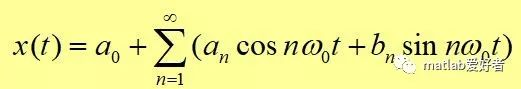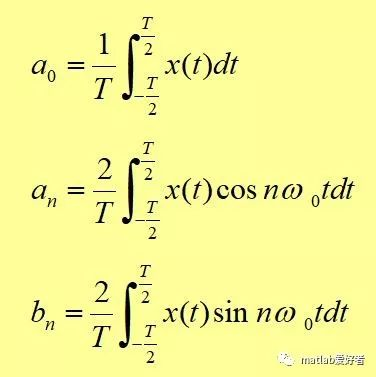以上是三角展开形式，今天我们不用这个，完全是为了方便大家理解，所以先说了一个简单的(见谅)。我们今天要用的是复指数形式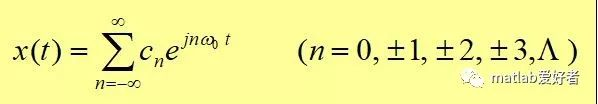当然，两种形式可以相互转换的，只需要利用欧拉公式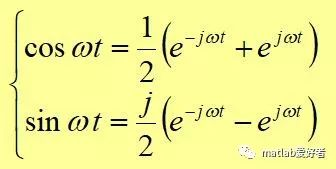下面，我举一个简单的函数作为例子f(t)=sin(t)+2*cos(t)。我们来对它进行傅里叶变换。根据已有的公式，我们一步步推进，我们先画出这个函数的图像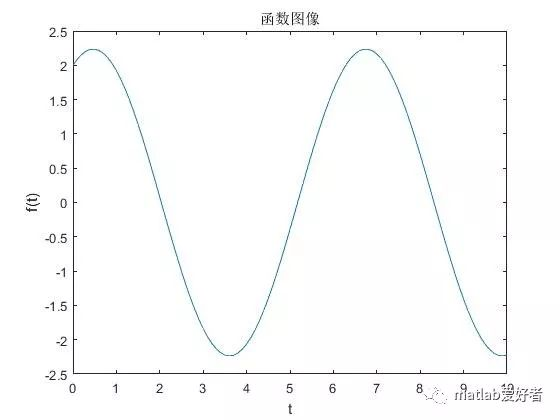下面的几步将傅里叶变换的参数全部求解出来，依据的就是复指数形式表达式(看不懂的同学倒回去看一看刚才讲的公式)。k=-N:N;W=pi*k/(N*dt);Fw=dt*ft*exp(-j*t1'*W);然后，对Fw进行一次绝对值变化。得到最终的结果。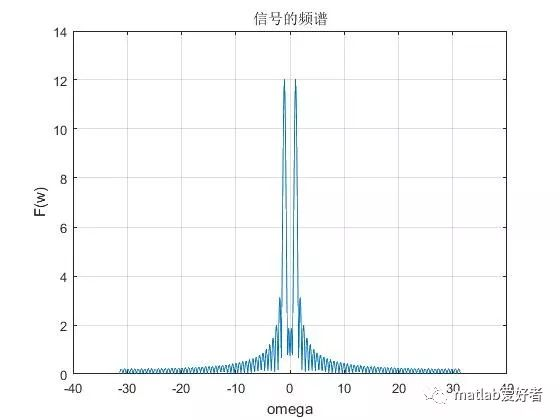以上就是信号的频域分析。即傅里叶表达式图像既然有频域函数，那当然就有频谱图。周期函数往往是连续的，但是频率却是离散的。废话不说了，上代码：Ts=0.4;  %采样周期,可通过改变采样周期大小观察抽样信号的频谱变化dt=0.1;t1=0:dt:10;ft=sin(t1)+2*cos(t1);%subplot(1)plot(t1,ft);%gridaxis('auto');xlabel('t');ylabel('f(t)');title('函数图像');hold onfigureN=400;k=-N:N;W=pi*k/(N*dt);Fw=dt*ft*exp(-j*t1'*W);plot(W,abs(Fw));grid;axis('auto')xlabel('omega');ylabel('F(w)');title('信号的频谱');hold onfigure%下面代表抽样过程t2=0:Ts:10;fst=sin(t2)+2*cos(t2);plot(t1,ft,'r*');hold onfigure%stem适合画火柴棍型图stem(t2,fst);gridaxis('auto')xlabel('t');ylabel('fs(t)');title('抽样后的信号');%hold offFsw=Ts*fst*exp(-j*t2'*W);%subplot(224)plot(W,abs(Fsw));gridaxis('auto')xlabel('omega');ylabel('Fs(w)');title('抽样信号的频谱');

展开全文• 快速傅里叶变换函数（fft)matlab编写
matlab 快速傅里叶变换函数（fft)编写（横向读取数据进行变换）
（欢迎指正）
参考资料百度文库PPT链接： https://wk.baidu.com/view/da8d58d776a20029bd642d14?pcf=2
直接甩代码
#matlab代码
#%快速傅里叶变换，不够2的整数幂的个数，末尾自动补齐0
function ret_val = myfft(Vector)
%因为输入的数据可能不是2的整数次幂，变换使得计算更加方便
[m,n]=size(Vector);%输入信号矩阵大小
num=ceil(log2(n));%向上取整
N=2^num;
vector=zeros(m,N);%申请足够大小矩阵
vector(:,1:n)=Vector(:,:);%将变换后的信号输入，不足补零
%变址运算
% temp=zeros(1,N);
% for p=1:m
%     for q=0:N-1
%         t=bin2dec(fliplr(dec2bin(q,num)));%变址运算
%         temp(1,q+1)=vector(p,t+1);
%     end
%     vector(p,:)=temp(1,:);
% end
%变址运算
for line=1:m%循环行数
j1 = 0;
for i = 1 : N%循环个数
if i < j1 + 1
tmp = vector(line,j1 + 1);
vector(line,j1 + 1) = vector(line,i);
vector(line,i) =tmp;
end
k = N / 2;
while k <= j1
j1 = j1 - k;
k = k / 2;
end
j1 = j1 + k;
end
end
for lines=1:m%循环行数
for L=0:num-1%L表示运算等级或者层数
dis=2^L;%dis表示奇偶组之间的距离
for id=1:2^(num-L-1) %循环当前层数组数
%进行同址运算
for idx=1:dis%循环组内个数
x1=(id-1)*2*dis+idx;%求得奇数数组的索引值
x2=(id-1)*2*dis+dis+idx;%对应偶数数组的索引值
temp1=vector(lines,x1)+vector(lines,x2)*W(L,(x1-1));%中间变量保存相应奇偶数组数据
temp2=vector(lines,x1)-vector(lines,x2)*W(L,(x1-1));
vector(lines,x1)=temp1;%所谓存入之前地址
vector(lines,x2)=temp2;
end
end
end
end
ret_val =vector;
function val=W(L,x)%旋转因子当层数为L,索引值为x
val=exp(-1j*2*pi*x/2^(L+1));
end
end

输入遥感影像数据进行测试结果截图*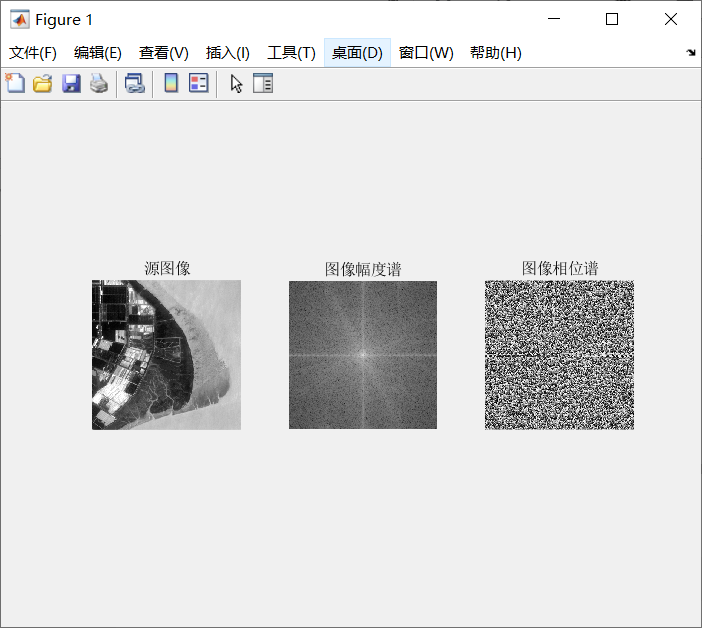输入矩阵或者数组进行测试并和matlab自带函数比较截图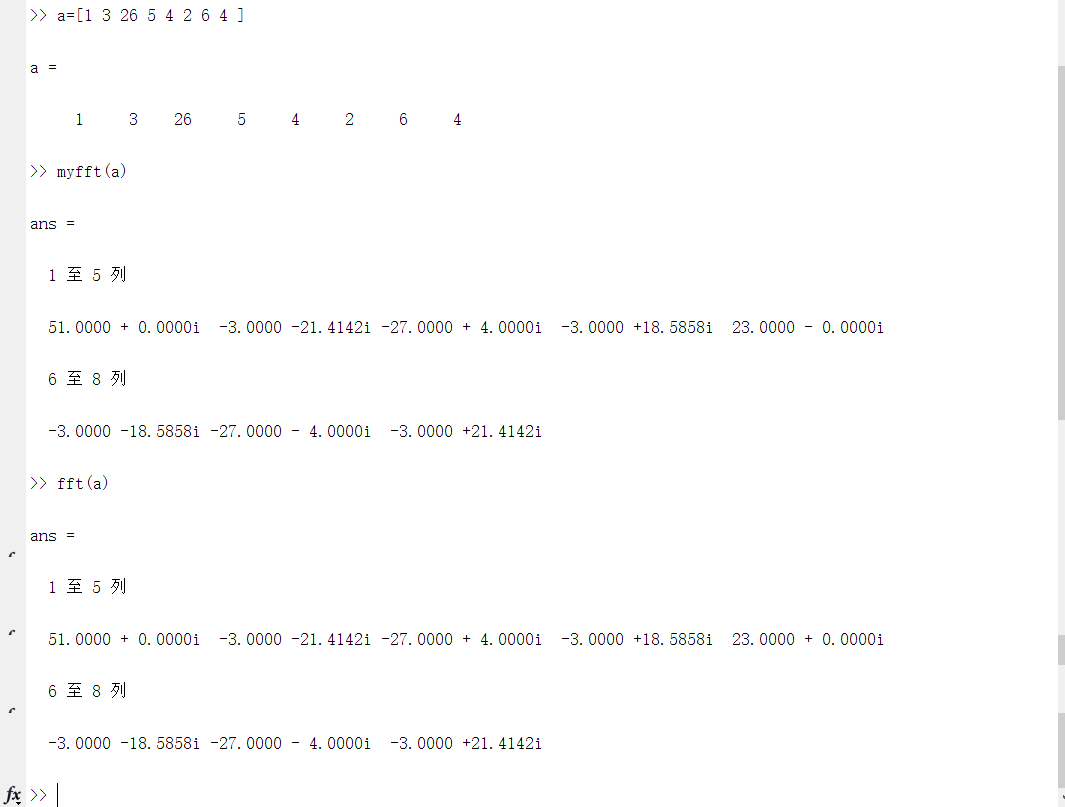需要注意的是自己编写的myfft的横向读取数据和自动补零的操作方式和matlab自带fft函数有区别，不完全相同。
具体运行代码：


展开全文• 满意答案fangong19782013.09.10采纳率：56%等级：...下面介绍一下用法格式:[tfr, t, f] = tfrstft(x) % 计算时间序列x的短时傅里叶变换，参数tfr为短时傅里叶变换系数，t为系数tfr对应的时刻，f为归一化频率向量[tfr...
满意答案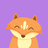fangong19782013.09.10采纳率：56%    等级：11已帮助：5250人首先，我的答案是针对于matlab时频分析工具箱的，在这个工具箱里，段师傅里叶变换的函数为tfrstft 。下面介绍一下用法格式:[tfr, t, f] = tfrstft(x) % 计算时间序列x的短时傅里叶变换，参数tfr为短时傅里叶变换系数，t为系数tfr对应的时刻，f为归一化频率向量[tfr, t, f] = tfrstft(x, t) % 计算对应时刻t的短时傅里叶变换[tfr, t, f] = tfrstft(x, t, n) % 计算n点对应时刻t的短时傅里叶变换[tfr, t, f] = tfrstft(x, t, n, h) % 参数h为归一化频率平滑窗[tfr, t, f] = tfrstft(x t, n, h, trace) % trace显示算法进程说明:x--信号t--时间(缺省值为1:length(x))n--频率数(缺省值为length(x))h--频率滑窗，h归一化为单位能量(缺省值为hamming(n/4))trace--如果非零，显示算法的进程(缺省值为0)tfr--时频分解(为复值)，频率轴观察范围为-0.5~0.5也就是说，如果你想改变窗函数，你需要修改h这个变量，希望对你有帮助11分享举报
展开全文• 快速计算傅里叶变换matlab函数快速计算傅里叶变换matlab函数快速计算傅里叶变换matlab函数快速计算傅里叶变换matlab函数快速计算傅里叶变换matlab函数快速计算傅里叶变换matlab函数快速计算傅里叶变换的...
• 傅里叶变换与信号频谱图MATLAB 仿真实验傅里叶变换与信号频谱图本实验将简要介绍如何利用FFT 函数描绘指定信号的频谱图像。一、相关函数1、FFT 函数离散傅里叶(Fourier )变换函数。【语法】Y = fft(X)Y = fft(X,n)Y ...
• 基于Matlab的二维快速傅里叶函数，将此程序放到同一文件夹内可直接调用！
• BB： 最近做脑电信号的时频分析，考虑采用该函数，但在参数设置和输出结果上有些疑惑，参考了众多资料，现对出现的困惑进行一些总结。 如何理解spectrogram()函数的输入输出参数？ Abstract: 我想这篇博文可以帮你弄...信号处理
• 函数形式： spectrogram support documentsc++ 开发语言 c语言 python
• 傅里叶变换是一种线性积分变换，用于信号在时域（或空域）和频域之间的变换，在物理学和工程学中有许多应用。1.傅里叶级数先从傅里叶级数讲起，...连续傅里叶变换将可积函数 f(t) 成 复指数函数的积分或级数形式。...
• FFT（Fast Fourier Transformation）为一阶快速傅里叶变换函数，在数字信号处理中有着广泛的应用，变换结果为复数。有些信号在时域上很难看出变化特征，但如果变换到频域之后，就很容易看出特征了。FFT把时域信号...fft
• 在此基础上进行推广，从而可以对一个非周期函数进行时频变换。从分析的角度看，他是用简单的函数去逼近(或代替)复杂函数，从几何的角度看，它是以一族正交函数为基向量，将函数空间进行正交分解，相应的系数即为坐标...
• MATLAB中想要画一个sin函数是很容易的。比如:首先定义t = 0:0.01:10,然后画出y = sin(2*pi*t)就可以了，最多再加相角啊之类的参数。但如果在C语言中应该如何自己制造一个sin函数呢？应该借鉴数字信号处理里的抽样...
• 文章目录傅里叶变换及傅里叶逆变换定义窗函数/矩形脉冲信号的傅里叶变换基于MATLAB的快速傅里叶变换FFT 傅里叶变换及傅里叶逆变换定义 能从时域的非周期连续信号转化到频域非周期连续信号。 窗函数/矩形脉冲...
• 傅里叶变换matlab FFT 函数解析 傅里叶变换网上有很多资料了，本博客主要讲实现《信号系统》P181页，不同周期的方波 的傅里叶级数： 与sinc形成包络： 其间隔ω0，如下图： 本博客用matlab 实现一下。 下面...信号处理
• 只分析一维度的 ...正变换： fftw_complex *in = fftw_malloc ( sizeof ( fftw_complex ) * n ); fftw_complex *out = fftw_malloc ( sizeof ( fftw_complex ) * n ); plan_forward = fftw_plan_dft_1d ...
• 今天，二狗给大家讲一讲Matlab实现傅里叶变换。大家都知道，信号分为两种，确定信号和不确定信号。在确定信号中，有两个非常重要的类别，时域分析和频域分析。而将两者充分结合的，就是我们今天要讲的傅里叶变换。绝...
• matlab 编写快速傅里叶变换函数（ifft）（横向读取数据进行变换） %快速傅里叶逆变换，不够2的整数幂的个数，末尾自动补齐0
• MATLAB中可以用fourier函数来算出信号的傅里叶变换 syms w f(t) f(t)=sinc(t); F1 = fourier(f(t), t, w) ezplot(F1,[-10*pi,10*pi],1)
• 话题：用MATLAB如何实现傅里叶变换问题详情：任意输入一个函数,然后,输出函数的傅里叶变换函数,然后回答：x=sin(2*pi*t);%任意输入一个函数 y=fft(x);%傅里叶变换函数 plot(abs(y));%振幅频率话题：matlab编程实现傅...
• 使用matlab中自带的fft等相关的函数进行操作。代码如下：clear;clc;img=imread('test.jpg');... %傅里叶变换f=fftshift(f); %使图像对称r=real(f); %求图像频域实部i=imag(f); %求图像频域虚部margin=log...
• 傅里叶变换进行音频处理2012年第 1期 福 建 电 脑 61比较在时间缩放和俯仰平移算法中海明窗函数和布菜克曼窗函数的效果林志伟 ，达 力 ，王 浩 ，韩 炜 ，林 凡(1．厦 门大学 计算机科学系 福建 厦 门 361005 2．......

# matlab傅里叶变换函数matlab 订阅